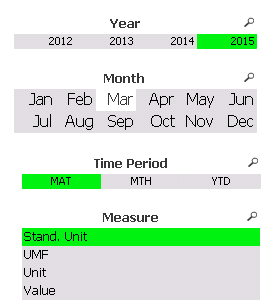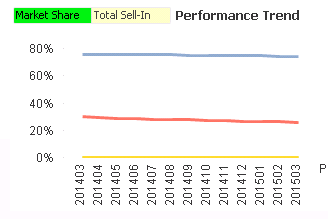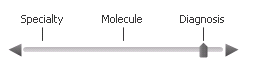# New to QlikView

Discussion board where members can get started with QlikView.

HighlightedNot applicable

## Monthwise Market Share Filter

Hello Everyone,

I need some help regarding a Month selection in my qvw file.

In my file, there are four filters (Year,Month, Time Period and Measure) available.
Please have a look at the below two screenshots.
The Requirement is, if I select Year (e.g 2015) , Month(Mar), Time Period (Mat) and Measure (Stand. Unit) then my chart should show latest 12 Months' Market Share.But the Chart Dimension is not a Date Column (e.g 201503, 201405 etc.).

What should be the expression? currently this expression is used:

Sum(CValue)

/

Sum(total CValue).

Looking forward to the correct answer.

Regards,

Sajid.

Tags (2)
6 RepliesMVP

## Re: Monthwise Market Share Filter

Hi,

Use calculated Dimension,

or

Regards

Great dreamer's dreams never fulfilled, they are always transcended.Not applicable

## Re: Monthwise Market Share Filter

Hello Max Dreamer,

We have faced similar problems in Quarterly Periods. Then we solved this using the below solution:

Data:

*,

MakeDate(Year, Right(Quarter, 1) * 3) AS Date

FROM DataSource;

You can also see the below thread for the previous solution.

QlikView Quarter Filter

But it was for Quarter Periods. Could you please tell us how we can do the same for Month Periods?

Best Regards,
Sajid.MVP & Luminary

## Re: Monthwise Market Share Filter

HI,

Try like this, arrive a date field in script and using that you can easily achieve this

Data:

*,

Date(Date#(Date, 'YYYYMM)) AS Date_Formatted

FROM DataSource;

Now in expression use this

=sum({<Year=, Quarter=, Date={'>=\$(=MonthStart(Max(Date_Formatted), -11))<=\$(=MonthEnd(Max(Date_Formatted)))'}>} MeasureName)

Hope this helps you.

Regards,

Jagan.Not applicable

## Re: Monthwise Market Share Filter

Hello Jagan,

As you can see that, this is for monthly periods, and in the below expression, there are Year and Quarter selected, but we have 4 filters in our scenario. Year, Month, TimePeriod and Measure. So, what should be the selections there?

=sum({<Year=, Quarter=, Date={'>=\$(=MonthStart(Max(Date_Formatted), -11))<=\$(=MonthEnd(Max(Date_Formatted)))'}>} MeasureName).

Another thing is, how can we calculate the Market Share? It should be like below:

Sum(CValue)

/

Sum(total CValue).

But I need a expression for this with the selections and with 12 months.

On top of that, the below load script is designed for external data source:

Data:

*,

Date(Date#(Date, 'YYYYMM)) AS Date_Formatted

FROM DataSource;

But how can I create the Date(Date#(Date, 'YYYYMM)) in sql? in our scenario, we are fetching data directly from sql database.

Best Regards,

Sajid.MVP & Luminary

## Re: Monthwise Market Share Filter

Hi,

Change the script like below if you are using SQL, I just given for sample purpose

Data:

*,

Date(Date#(Date, 'YYYYMM)) AS Date_Formatted;

SELECT

*

FROM YourSQLTableName;

Now use below expression

Sum({<Year=, Month=, TimePeriod=, Date_Formatted={'>=\$(=MonthStart(Max(Date_Formatted), -11))<=\$(=MonthEnd(Max(Date_Formatted)))'}>} CValue)

/

Sum({<Year=, Month=, TimePeriod=, Date_Formatted={'>=\$(=MonthStart(Max(Date_Formatted), -11))<=\$(=MonthEnd(Max(Date_Formatted)))'}>} total CValue).

Hope it helps you.

Regards,

Jagan.Not applicable

## Re: Monthwise Market Share Filter

Hi Jagan:

We have tried to implement the changes. But still facing problems to calculate Market Share.

We actually skipped one important thing to mention which is slider in our dashboard which is like below:You can see that there are 3 items in the slider which are Specialty, Molecule and Diagnosis. Do you think this is the main reason our Market Share is not returning the correct value?

I don’t know how to handle expression when I use slider.

In the related chart we have taken the following dimensions with conditions and expression:

Chart:Dimension1: Specialty, corresponding condition: SubStringCount(Concat(_MADimension, '|'), 'Specialty')

Dimension2: Molecule, corresponding condition: SubStringCount(Concat(_MADimension, '|'), ‘Molecule’)

Dimension3: Diagnosis

, corresponding condition: SubStringCount(Concat(_MADimension, '|'), ‘Diagnosis’)

Dimension4: Date

Measure Expression: =sum({<MAYEAR=,MAQUARTER=, Date={'>=\$(=QuarterStart(Max(Date), -7))<=\$(=QuarterEnd(Max(Date)))'}>}Proj__Default_Crp)
/
sum({<MAYEAR=,MAQUARTER=, Date={'>=\$(=QuarterStart(Max(Date), -7))<=\$(=QuarterEnd(Max(Date)))'}>}total Proj__Default_Crp

It would be nice if you could help me in this regard.

Thanks a lot and Best Regards,

Sajid